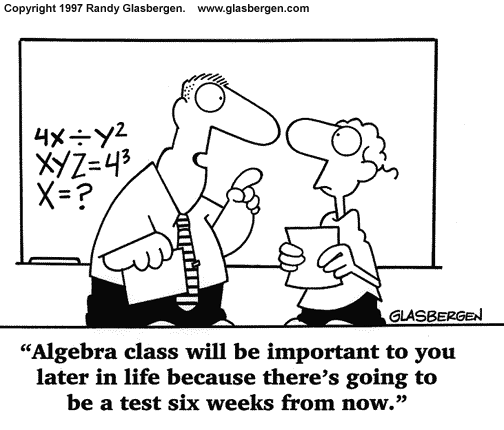## Equations & InequationsNew Topic: Equations & Inequations, MiA 4³ Ch5

By the end of this topic you will have revised your basic algebra skills including:

• gathering like terms & simplifying expressions
• substituting values (including negative numbers)
• evaluating expressions
• multiplying out brackets (single and double)
• solving equations
• You will be able to solve:

• equations involving expanding double brackets
• equations with fractions
• inequations ( etc)
• ## Equations and InequationsNew Topic: Equations & Inequations, MiA 4² Ch6

By the end of this topic you will be able to solve equations involving variables on both sides, equations with brackets and inequalities.